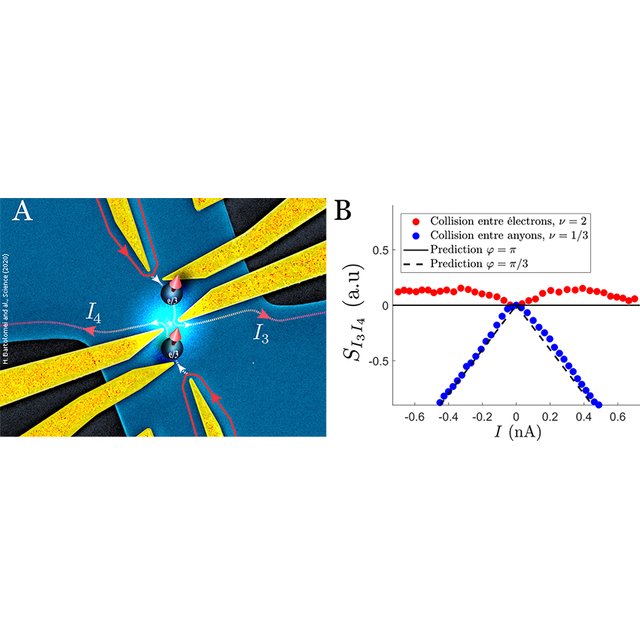News

Published the April 10, 2020

Fractional statistics of anyons in a two-dimensional conductorBy implementing a collider experiment between the elementary excitations of a two-dimensional conductor, physicists have revealed the fractional statistics of anyons, interpolating between fermionic and bosonic statistics. These results could open the way to topological quantum computing based on the exchange of some varieties of anyons.

In three-dimensional space, elementary particles are divided exclusively between fermions (like electrons for example) and bosons (like photons) according to the properties of symmetry of the wave function describing the state of the system when two particles are exchanged. When exchanging two fermions, the wave function acquires a phase, φ = π. On the other hand, in the case of bosons, this phase is zero, φ = 0. This difference leads to deeply distinct collective behaviors between fermions, which tend to exclude themselves, and bosons which tend to bunch together. The situation is different in two-dimensional systems which can host exotic quasiparticles, called anyons, that obey intermediate quantum statistics characterized by a phase φ varying between 0 and π. The existence of these quasiparticles was predicted, almost forty years ago, in two-dimensional conductors where the correlations between electrons are very strong, like for example in the quantum Hall regime for example.

This quantum Hall regime is obtained by applying a magnetic field perpendicular to the surface of a two-dimensional electron gas. In a quantum description of the system, the kinetic energy of the electrons is quantized and, for strong magnetic fields (typically around ten Tesla), all the electrons occupy a single energy level. The kinetic energy is then frozen and the interactions between electrons play a dominant role, resulting in very strong correlations between electrons which favor the emergence of these exotic quasiparticles. In the fractional quantum Hall regime, reached when a fraction υ = 1 / m (m = 3, 5, …) of the first kinetic energy level is occupied, the elementary excitations carry a fractional charge q = e / m and have been predicted to obey fractional statistic characterized by an exchange phase φ = π / m. Their fractional charge has been observed but, despite numerous studies over the past thirty years, no clear experimental evidence of fractional statistics has been obtained so far.

By implementing and studying anyon collisions in a two-dimensional electron gas, physicists from the Physics Laboratory of Ecole Normale Supérieure (LPENS) and from the Centre de Nanosciences et de Nanotechnologies (C2N) have demonstrated the fractional statistics of anyons. Their work has been published in Science and featured on the cover of this week's issue.

The collider, fabricated at C2N (figure A) is a small electronic chip (with a typical size of a few microns) designed in a high mobility two-dimensional electron gas in a GaAs/AlGaAs heterostructure. Metallic gates deposited at the surface of the electron gas and called quantum point contacts (QPC) can be used to randomly transmit quasiparticles. Two QPC’s (QPC1 and QPC2 in the figure) emit quasiparticles towards the QPC located at the center of the sample (cQPC in the figure) and used as the beam-splitter in the collision. The experiments were performed at LPENS in a dilution fridge allowing the researchers to cool down the chip to a few tens milliKelvins which is necessary to reach the fractional quantum Hall regime. The collisions were first studied in the integer quantum Hall regime (when the number υ of occupied energy levels is an integer, here υ=2). In this regime, the elementary excitations are electrons which obey the usual fermionic statistics. It leads to the observation of an antibunching effect in an electron collision: electrons systematically exit in two different arms of the beam-splitter. This effect, related to the Pauli exclusion principle, can be revealed by measuring the cross-correlations of the current fluctuations at the output of the splitter (Figure B). The fermionic statistics leads to their complete suppression. The observed result is completely different for a fractional filling υ=1/3. Fractional statistics leads to a suppression of the antibunching effect and quasiparticles tend to bunch together in larger packets of charge in a single output of the splitter. This effect leads to the observation of negative correlations of the current fluctuations (Figure B) in perfect agreement  with recent theoretical predictions for an exchange phase φ=π/3 expected for υ=1/3.

These experiments confirm the combined roles of strong correlations and low dimensionality for the emergence of anyons with statistics intermediate between those of electrons and bosons. They could be generalized, in the future, to other fractional fillings of the first energy level (like υ=2/3,1/5,…) for which other fractional statistics are expected. In particular, implementing these experiments in the non-abelian regime (for filling υ=5/2 for example), where exchange operations do not commute and thus cannot be described by a simple phase would open the way to topological quantum computing based on the braiding of these exotic quasiparticles.

References:
Fractional statistics in anyon collisions
H. Bartolomei1, M. Kumar1, R. Bisognin1, A. Marguerite1, J.-M. Berroir1, E. Bocquillon1, B. Plaçais1, A. Cavanna2, Q. Dong2, U. Gennser2, Y. Jin2 et G. Fève1
Science, Vol. 368, Issue 6487, pp. 173-177 (2020)
DOI : 10.1126/science.aaz5601

1 Laboratoire de Physique de l’Ecole normale supérieure - LPENS (ENS, Université PSL, CNRS, Sorbonne Université, Université Paris-Diderot)
2 Centre de Nanosciences et de Nanotechnologies – C2N (CNRS, Université Paris-Saclay), Palaiseau

Contacts:

Figure A: Scanning electronic microscope image (in false colors) of the anyon collider. The two-dimensional electron gas is in blue. Quasiparticles move along the edge channels (in red). Pairs of electrodes (in gold) define beam-splitters called quantum point contacts (QPC). QPC1 and QPC2 emit quasiparticles colliding on cQPC. Figure B: measurements of the correlations Si3i4 of the fluctuations of the currents i3 and i4 at the output of the beam-splitter as a function of the incoming quasiparticle current.